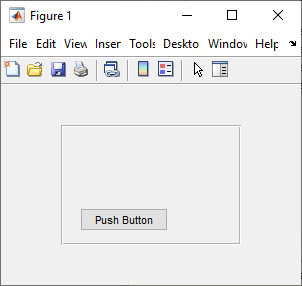# getpixelposition

Get component position in pixels

## Syntax

``pos = getpixelposition(c)``
``pos = getpixelposition(c,isrecursive)``

## Description

example

````pos = getpixelposition(c)` returns the position in pixels of the component specified by `c`. MATLAB® returns the position as a four-element vector that specifies the location and size of the component relative to its parent container in the form ```[left bottom width height]```.```

example

````pos = getpixelposition(c,isrecursive)`, where `isrecursive` is `true`, returns the component position relative to the parent figure. The default for `isrecursive` is `false`, which returns the position relative to its immediate parent container.```

## Examples

collapse all

Create a push button within a panel container.

```f = figure('Position',[300 300 300 200]); p = uipanel('Position',[.2 .2 .6 .6]); btn = uicontrol(p,'Style','PushButton', ... 'Units','Normalized', ... 'String','Push Button', ... 'Position',[.1 .1 .5 .2]);```Get the position in pixels of the push button relative to its parent container, the panel.

```pos = getpixelposition(btn) ```
```pos = 18.6000 12.6000 88.0000 23.2000```

Create a push button within a panel container.

```f = figure('Position',[300 300 300 200]); p = uipanel('Position',[.2 .2 .6 .6]); btn = uicontrol(p,'Style','PushButton', ... 'Units','Normalized', ... 'String','Push Button', ... 'Position',[.1 .1 .5 .2]);```Get the position in pixels of the push button relative to its parent figure.

```pos = getpixelposition(btn,true) ```
```pos1 = 78.6000 52.6000 88.0000 23.2000```

## Input Arguments

collapse all

UI component.

Whether to return the position relative to the parent figure, specified as a numeric or logical `0` (`false`) or `1` (`true`).

• `0` (`false`) — The function returns the component position relative to its immediate parent.

• `1` (`true`) — The function returns the component position relative to the figure window.

collapse all

### Pixels

Distances in pixels are independent of your system resolution on Windows® and Macintosh systems:

• On Windows systems, MATLAB defines a pixel as 1/96th of an inch.

• On Macintosh systems, MATLAB defines a pixel as 1/72nd of an inch.

On Linux® systems, your system resolution determines the size of a MATLAB pixel. For more information, see DPI-Aware Behavior in MATLAB.